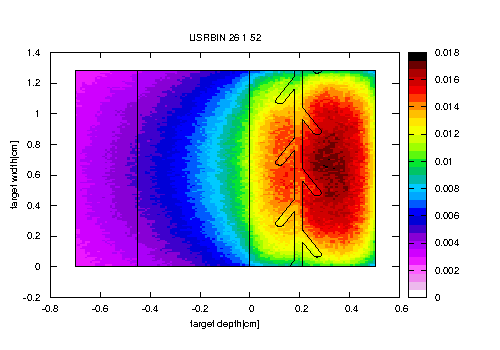# The unit of quantity scored by MGDRAW

Dear FLUKA experts,

My simulation is a proton beam incident on a metal target(there exist water channels inside target).
I want to get the information about the positions of (p,n) reactions, so I use a customized mgdraw (usdraw entry) user routine to record the coordinates(x,y,z) of positions where (p,n) reactions take place.

and I used the coordinates (y,z) to get a figure (2D distribution) which shows the positions where (p, n) reactions take place in y(target width)-z(target depth) transversal area (as shown in

). All the red points represent the positions where neutrons produced by (p, n). For this I’d like to know the unit of this quantity or event?
And in order to evaluate neutron yield which produced by (p,n) reactions along target depth, I consider the number of (p,n) reactions as the amount of neutrons produced by (p,n). So I integrated the number of (p,n) reactions and get the number of reactions(neutrons) in different target depth (like ). For my understanding the values at y-direction mean the number of neutrons produced by (p,n) reactions, and the unit should be [1/cm]. could you please tell me is it right?

The above is my personal understanding, if there is anything wrong, please don’t hesitate to correct me.

Any comments or suggestions will be appreciated.

Best regards,

Qi Ding

Hallo @qding
the first figure is a scatter plot, where you are not plotting any physical quantity, rather the position of the reactions collapsed on a yz plane. To get some physical quantity out of that, you should count the number of reactions in arbitrary dydz bins and divide by the bin volume dxdydz, where dx is the considered x range, as well as by the number of simulated beam protons. Then you could do a color plot giving the reaction density (cm^-3) per beam proton, by associating to each dydz bin the respective result.
As for the second figure, since here you have counted the number of reactions in dy bins, you should divide by the considered dy step (cm) and again by the number of simulated beam protons and then - if indeed the scored reaction is a (p,n) one, as it’s up to you to check in usdraw - you will get the linear density (cm^-1) of produced neutrons in those reactions (integrated over the xz plane) per beam proton.
All in all, I’d find a standard 3D USRBIN scoring of neutron fluence much more straightforward and useful.

Dear Francesco,

thanks a lot for your detailed response.
this is my input file and magraw.f filepn.inp (13.4 KB) mgdraw.f (3.6 KB) .
In order to activates calls to the user routine MGDRAW, I put the USERDUMP card in the input file. This card does not define or divide scoring region into many small bins.
so if I want to count the number of reactions in arbitrary dydz bins, how do I divide the region into many small bins. And another question is you mentioned above " divide by the bin volume dxdydz, where dx is the considered x range", does the “dx” mean the total target length in x-direction or the size of a small bin?
and also regarding USRBIN scoring card, I got neutron fluencewith this card, but for my understanding it’s only show the neutron track length density. My aim is to get the number of neutrons produced by (p,n) reaction and the reaction density.
Is there a way to get these information with USRBIN card?

Best regards,

Qi Ding